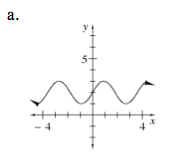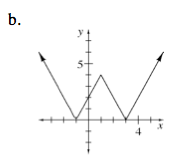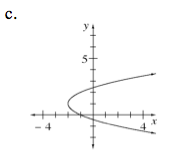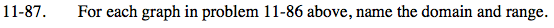Home > AC > Chapter 11 > Lesson 11.2.1 > Problem11-87

11-87.

For each graph in problem 11-86 above, name the domain and range. Homework Help ✎To find the domain of each graph, find the possible x-values.

To find the range of each graph, find the possible y-values.

Domain: All real numbers
Range: y ≥ 0

Either the domain or the range is the set of all real numbers.